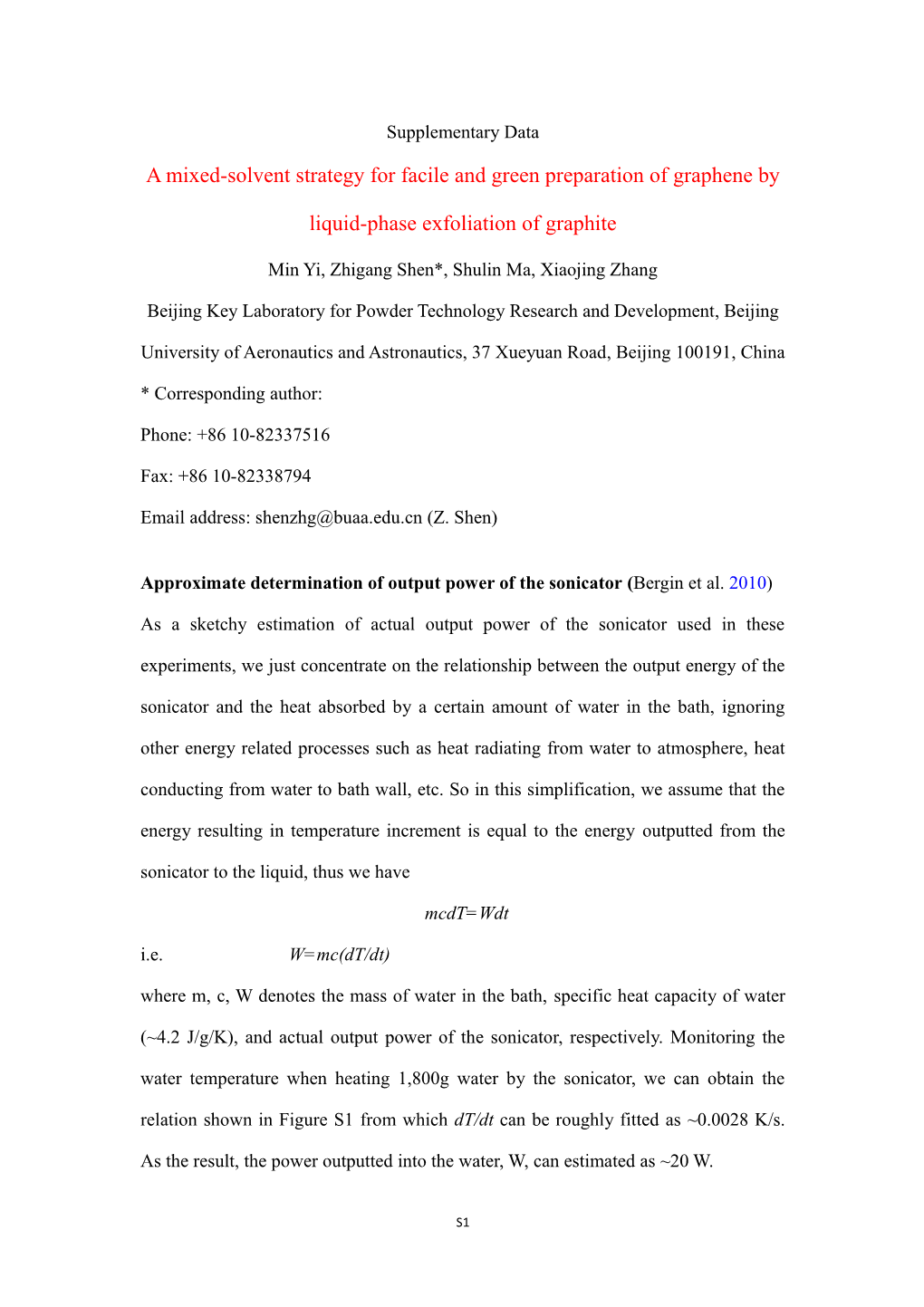# Min Yi, Zhigangshen*, Shulin Ma, XiaojingzhangSupplementary Data

A mixed-solvent strategy for facile and green preparation of graphene by liquid-phase exfoliationof graphite

Min Yi, ZhigangShen*, Shulin Ma, XiaojingZhang

Beijing Key Laboratory for Powder Technology Research and Development, BeijingUniversity of Aeronautics and Astronautics, 37 Xueyuan Road, Beijing 100191, China

* Corresponding author:

Phone: +86 10-82337516

Fax: +86 10-82338794

### Approximate determination of output power of the sonicator (Bergin et al. 2010)

As a sketchy estimation of actual output power of the sonicator used in these experiments, we just concentrate on the relationship between the output energy of the sonicator and the heat absorbed by a certain amount of water in the bath, ignoring other energy related processes such as heat radiating from water to atmosphere, heat conducting from water to bath wall, etc. So in this simplification, we assume that the energy resulting in temperature increment is equal to the energy outputted from the sonicator to the liquid, thus we have

mcdT=Wdt

i.e. W=mc(dT/dt)

where m, c, W denotes the mass of water in the bath, specific heat capacity of water (~4.2 J/g/K), and actual output power of the sonicator, respectively. Monitoring the water temperature when heating 1,800g water by the sonicator, we can obtain the relation shown in Figure S1from which dT/dtcan be roughly fitted as ~0.0028 K/s. As the result, the power outputted into the water, W, can estimated as ~20 W.

Figure S1. Temperature (T) as a function of time (t)

Table S1 HSP and density of substances studied here*(Hernandez et al. 2010; Hansen 2007)

Substance / D(MPa1/2) / P(MPa1/2) / H(MPa1/2) / (g/cm3)
Water / 18.1 / 17.1 / 16.9 / 1
Ethanol / 15.8 / 8.8 / 19.4 / 0.79
Isopropanol / 15.8 / 6.1 / 16.4 / 0.785
Graphene / 18 / 9.3 / 7.7 / —

*According to Hansen (Hansen 2007), the HSP of a substance can be divided into dispersion component (D), dipolar component (P), and hydrogen-bonding component (H). The HSP of abinary mixture is proportional to the volume fraction of each component, i.e. i,mix=(m1/1i,1+m2/2i,2)/(m1/1+m2/2)where i=D, P ,H and mj,j (j=1,2) is the mass fraction and density of solvent j, respectively. Thus, for Water-Ethanol mixtures (W-E), RaW-E=(4(D,W-E-D,Graphene)2+(P,W-E -P,Graphene)2+(H,W-E -H,Graphene)2)1/2, and for Water-Isopropanol mixtures (W-IPA), RaW-IPA=(4(D,W-IPA-D,Graphene)2+(P,W-IPA -P,Graphene)2+(H,W-IPA -H,Graphene)2)1/2. The HSPs of water, ethanol and isopropanol were taken from the Hansen handbook (2007), while the HSP of graphene was from Hernandez etal (2010).The HSP of water used in the manuscript are based on a correlation of complete miscibility, as reported on page 22 of the Hansen handbook (2007).Figure S2. Details about fitting sedimentation curves where C0/CI=y0, =t1. The exponential decay fitting in a suspension system is characterized by stable phase (concentration C0) and sedimenting phase (concentration CI-C0, where CI isinitial concentration).

Table S2Fitting parameters of sedimentation curves from Figure S2

Parameters / W-E,1Krpm / W-IPA,1Krpm / W-E,3Krpm / W-IPA,3Krpm
C0/CI / 67.42%±0.5% / 80.23%±0.7% / 93.19%±0.3% / 90.04%±0.5%
 / 128.7±6.3 h / 138.8±15.4 h / 158.1±21.1 h / 74.06±13.6 h
R2 / 0.99693 / 0.9844 / 0.98091 / 0.94331
Chi2/DoF / 0.00004 / 0.00009 / 0.00001 / 0.00008

Figure S3. Typical AFM images and height profiles of graphene deposited from W-E dospersion.

Figure S4. Some typical AFM images and height profiles of graphene deposited from W-IPA dospersion.

### References

Bergin SD, Sun Z, Streich PV, Hamilton JP, Coleman JN (2010) New solvents for nanotubes-approaching the dispersibility of surfactant. J Phys Chem C 114:231-237

Hernandez Y, Lotya M, Richard D, Bergin SD, Coleman JN (2010) Measurement of multicomponent solubility parameters for graphene facilitates solvent discovery.Langmuir 26:3208-3213

Hansen CM 2007Hansen solubility parameters: A user’s handbook2nd ed (Boca Raton: CRC Press)

S1